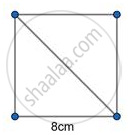# Find the Length of Diagonal of the Square Whose Side is 8 Cm. - Geometry

Find the length of diagonal of the square whose side is 8 cm.

#### SolutionLength of the Diagonal sqrt2 xx "side"

⇒ sqrt 2xx8

8sqrt2cm

Concept: Right-angled Triangles and Pythagoras Property
Is there an error in this question or solution?